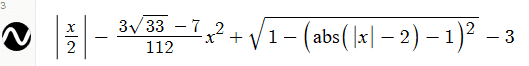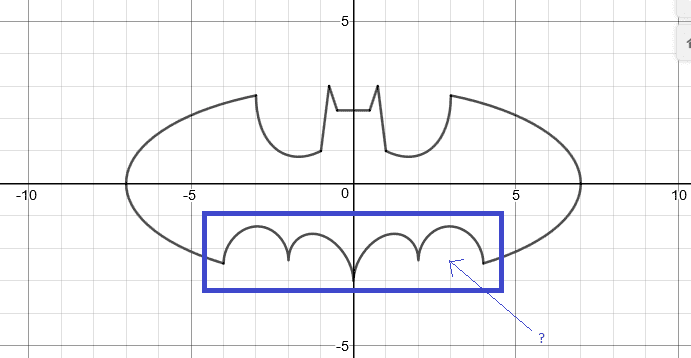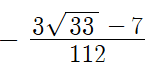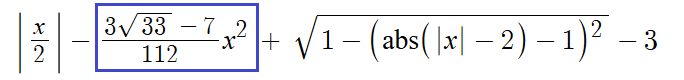# Purpose of coefficients in a equation or expression?

Hello.
I have an equation which I understand partially but after understanding the purpose of coefficients in the below equation I think my understanding will enhance.* I found it on: https://www.desmos.com/calculator/dnzfajfpym

What decides that I need coefficients for x2 in the given equation of this post?

I think that coefficients of x2 in this post's equation exist to scale up or scale down the size of the thing drawn in blue box: Correct?But how do I come up a number that is so precise like:which is the coefficient of x2 to complete the sketching of the curve (Batman)?

Do I need to solve a few equations to come up with the coefficient of x2 in the following equation?Thanks!

#### Attachments

•jedishrfu